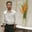Related Tags

php

# How to perform two way encryption using PHPBehzad Ahmad

## Overview

When we apply both the encryption and decryption functions to some data, we call it two-way encryption. In PHP, we can use the following two functions to perform two-way encryption.

• base64_encode()

• base64_decode()

### The base64_encode function

We can use the base64_encode function to encode the given data or string with the base 64.

string base64_encode ( string $array )  ### Parameters • array: This is the array or string we need to encode. ### Return type This function will return the encoded data of a given string. ### Example <?php$arr = "Encrypted String";
$temp = base64_encode($arr);
echo $temp; ?>  Example of encoding ### Explanation • Line 2: We declare a string and store data in it. • Line 3: We call the encryption function and pass the string to the function. • Line 4: We print the encrypted string. ### The base64_decode function We can use the base64_decode function to decode the base 64 encoded data. ### Syntax string base64_decode ( string$array)



### Parameters

• array: This is the data we need to decode.

### Return type

This function will return the decoded data.

### Example

<?php
$str = 'RW5jcnlwdGVkIFN0cmluZw=='; echo base64_decode($str);
?>  
Example of decoding

### Explanation

• Line 2: We declare a string and store encrypted data in it.

• Line 3: We call the decryption function and pass the string to the function.

• Line 4: We print the decrypted string.

RELATED TAGS

php

CONTRIBUTORBehzad Ahmad
RELATED COURSES

View all Courses

Keep Exploring

Learn in-demand tech skills in half the time